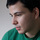enhancement## Array#intersect performance can be improved.

Reported by blatyo | September 10th, 2009 @ 07:54 PM

Before prototype 1.6.1, there was no Array#intersect, so I had written my own.

function intersect(array){
return this.without.apply(this, this.without.apply(this, array)); }

When prototype 1.6.1 came out I wrote up a test to compare the two. See that here:
Here are some of the results I got in milliseconds (running on chromium):

Testing My Intersect
4517
4614
4823
4998
4856

Testing Prototype's Intersect
7321
7337
7376
7353
7331

I have created a fork of prototype which contains this change and also the changes for ticket 793. This ticket depends on ticket 793, since currently without uses == comparison, while the current intersect uses === comparison. So ticket 793 changes without (along with others) to use === comparison.

My fork can be found here: http://github.com/blatyo/prototype/tree/master

### Comments and changes to this ticket

•#### T.J. CrowderNovember 16th, 2009 @ 04:50 PM

[responsible:none bulk edit command]

•#### Tisho GeorgievMarch 1st, 2010 @ 01:54 PM

• State changed from “new” to “enhancement”
•#### William WarbyMarch 27th, 2011 @ 07:31 PM

• Importance changed from “” to “Medium”

I hesitated to post this because I think I've come up with a version of Array#intersect that is comfortably over 1,000 times faster than the current version, which seems ridiculous. I'm sure I'll soon be told why my version is no good or how I'm using the current version wrong, but here goes...

``````//Utility method for generating a random string of a specified length
String.random = function(l) {
var ret = '';
for (var x = 0; x < l; x++) { ret += String.fromCharCode(Math.floor(Math.random() * 26) + 97); }
return ret;
};

//Alternative implementation of Prototype's Array#intersect using Array#uniq to guarantee uniqueness before intersecting
//If both the source and comparison arrays are already unique, set the argument "u" to a truthy value for extra performance
Array.prototype.fastIntersect = function(a, u) {
var x, r = [], b = u ? this.concat(a).sort() : this.concat([]).sort().uniq(true).concat(a.concat([]).sort().uniq(true)).sort();
for (x = 0; x < b.length; x++) {
if (b[x] == b[x - 1] && r[r.length - 1] !== b[x]) { r.push(b[x]); }
}
return r;
};

(function() {

var x, randomStrings1 = [], randomStrings2 = [];

/* Create two arrays each containing 2,000 x 4 character random strings for testing */
for (x = 0; x < 2000; x++) {
randomStrings1.push(String.random(4));
randomStrings2.push(String.random(4));
}

/* Average results for Firefox 4: ~7625ms */
console.time('Array#intersect (Prototype 1.7)');
console.log(randomStrings1.intersect(randomStrings2).length + ' matches');
console.timeEnd('Array#intersect (Prototype 1.7)');

/* Average results for Firefox 4: ~12ms */
console.time('Array.prototype.fastIntersect');
console.log(randomStrings1.fastIntersect(randomStrings2).length + ' matches');
console.timeEnd('Array.prototype.fastIntersect');

/* Average results for Firefox 4: ~1ms with pre-sorted arrays */
randomStrings1 = randomStrings1.sort().uniq(true);
randomStrings2 = randomStrings2.sort().uniq(true);
console.time('Array.prototype.fastIntersect (assume unique)');
console.log(randomStrings1.fastIntersect(randomStrings2, true).length + ' matches');
console.timeEnd('Array.prototype.fastIntersect (assume unique)');

})();```
```

The performance increase comes by sorting and concatenating the arrays and looking for duplicates in a single iteration rather than hunting for needles in haystacks as I believe the current version does. I've stress tested it by comparing two arrays of strings containing 1 million members each, and it can accurately identify the overlap in about 10 seconds.

•#### Dan PopescuMarch 31st, 2011 @ 08:44 AM

``````  Array.prototype.fastIntersect1 = function(a, u) {
var x, r = [], b = u ? this.concat(a).sort() : this.concat([]).sort().uniq(true).concat(a.concat([]).sort().uniq(true)).sort();
for (x = b.length; --x;) {
if (b[x] == b[x - 1] && r !== b[x]) { r.unshift(b[x]); }
}
return r;
};```
```
•#### Dan PopescuMarch 31st, 2011 @ 08:49 AM

sorry

``````  function fastIntersect(array, sorted) {
var r = [],
b = sorted ? this.concat(array).sort() : this.concat([]).sort().uniq(true).concat(array.concat([]).sort().uniq(true)).sort(),
x = b.length;
if (x > 0) {
for (; --x;) {
if (b[x] == b[x - 1] && r !== b[x]) { r.unshift(b[x]); }
}
}
return r;
}```
```
•#### Brian KennApril 21st, 2011 @ 12:39 AM

There's a minor bug here...

The Identity operator (===) should be used, not the Equality operator (==).

Here is the updated code...

``````function fastIntersect(array, sorted) {
var r = [],
b = sorted ? this.concat(array).sort() : this.concat([]).sort().uniq(true).concat(array.concat([]).sort().uniq(true)).sort(),
x = b.length;
if (x > 0) {
for (; --x;) {
if (b[x] === b[x - 1] && r !== b[x]) { r.unshift(b[x]); }
}
}
return r;
}```
```
•#### Shubham ThakurJanuary 19th, 2019 @ 01:28 PM

With your very own profile, you can contribute to projects, track your activity, watch tickets, receive and update tickets through your email and much more.